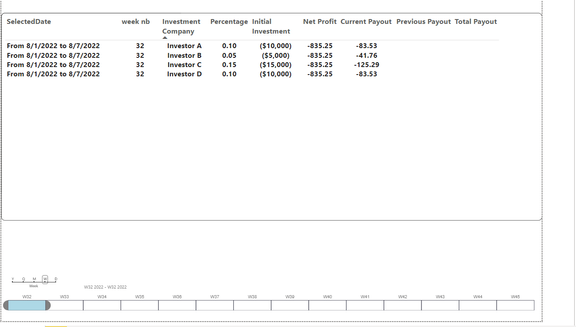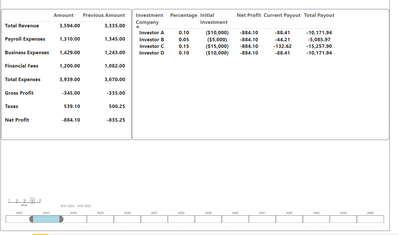cancel
Showing results for
Did you mean:Impactful Individual

## Incremental Values over period of time from calculated Measures

hello everyone, i am trying to build a table in pbix where it shows me payout of investors over a course of periods from my selected date, but i only have calculated measures for this case

you may find my pbix file here:
https://1drv.ms/u/s!Ag9tIyk2ofNRjkTpg8L4VKb3_Rzw?e=EBa9Wn

and a glimpse of my table how i want the design to be:and below, you can find my desired results and a walkthrough of how i want it calculated:

for the inital week (when investment was made) :and then for  selected period after( in my case week 2):1 ACCEPTED SOLUTIONImpactful Individual

i finally found a solution for me and i will guide you through the steps :

first i created a  net profit measure

``````Net Profit =
VAR Totalrevenue = Calculate(SUM(Revenue[Amount]))
VAR Payroll = CALCULATE(SUM(Expenses[Amount]),FILTER(Expenses,Expenses[Category] = "Payroll Expenses"))
VAR Financial = CALCULATE(SUM(Expenses[Amount]),FILTER(Expenses,Expenses[Category] = "Financial Fees"))
Var Totalexpense = Calculate(SUM(Expenses[Amount]))
Var Grossprofit = (Totalrevenue - Totalexpense)
VAR Taxes = (Totalrevenue * 0.15)
VAR Netprofit = Grossprofit - Taxes
Return
Netprofit``````

later i created a "before" measure that calculates all net profit from my inital date to selected date in the following measure :

``````before = VAR seldate = MAX(DateTable[Date])
RETURN
CALCULATE([Net Profit],DATESBETWEEN(DateTable[Date],"8/1/2022",seldate))``````

Then i made my current payout which is

``````Current Payout =
[Net Profit] * MAX(Investors[Percentage])``````

and finally i have the total payout which is :

``Total Payout = ([before] * MAX(Investors[Percentage])) + MAX(Investors[Initial Value])``

here are some photos of my results :
for week 1 :for week 2:all is left is for me to create a previous payout measure which i already know how to solve half of it i just need a similar calcualtion to the "before" measure but he seldate is 1 week before can anyone help?

Edit: i found a way to get previous payout
first i find the pre before:

``````pre before =
VAR _min =
MIN ( 'DateTable'[Date] )
VAR _Max =
MAX ( 'DateTable'[Date] )
VAR _days =
COUNTROWS ( CALENDAR ( _min, _Max ) )
VAR seldate = CALCULATE(MAX(DateTable[Date]),FILTER ( ALL ( DateTable ), [Date] <= _Max - _days && [Date] >= _min - _days ))
RETURN
CALCULATE([Net Profit],DATESBETWEEN(DateTable[Date],"8/1/2022",seldate))``````

then previous payout is:

``pre Payout = ([pre before] * MAX(Investors[Percentage])) + MAX(Investors[Initial Value])``Impactful Individual

i finally found a solution for me and i will guide you through the steps :

first i created a  net profit measure

``````Net Profit =
VAR Totalrevenue = Calculate(SUM(Revenue[Amount]))
VAR Payroll = CALCULATE(SUM(Expenses[Amount]),FILTER(Expenses,Expenses[Category] = "Payroll Expenses"))
VAR Financial = CALCULATE(SUM(Expenses[Amount]),FILTER(Expenses,Expenses[Category] = "Financial Fees"))
Var Totalexpense = Calculate(SUM(Expenses[Amount]))
Var Grossprofit = (Totalrevenue - Totalexpense)
VAR Taxes = (Totalrevenue * 0.15)
VAR Netprofit = Grossprofit - Taxes
Return
Netprofit``````

later i created a "before" measure that calculates all net profit from my inital date to selected date in the following measure :

``````before = VAR seldate = MAX(DateTable[Date])
RETURN
CALCULATE([Net Profit],DATESBETWEEN(DateTable[Date],"8/1/2022",seldate))``````

Then i made my current payout which is

``````Current Payout =
[Net Profit] * MAX(Investors[Percentage])``````

and finally i have the total payout which is :

``Total Payout = ([before] * MAX(Investors[Percentage])) + MAX(Investors[Initial Value])``

here are some photos of my results :
for week 1 :for week 2:all is left is for me to create a previous payout measure which i already know how to solve half of it i just need a similar calcualtion to the "before" measure but he seldate is 1 week before can anyone help?

Edit: i found a way to get previous payout
first i find the pre before:

``````pre before =
VAR _min =
MIN ( 'DateTable'[Date] )
VAR _Max =
MAX ( 'DateTable'[Date] )
VAR _days =
COUNTROWS ( CALENDAR ( _min, _Max ) )
VAR seldate = CALCULATE(MAX(DateTable[Date]),FILTER ( ALL ( DateTable ), [Date] <= _Max - _days && [Date] >= _min - _days ))
RETURN
CALCULATE([Net Profit],DATESBETWEEN(DateTable[Date],"8/1/2022",seldate))``````

then previous payout is:

``pre Payout = ([pre before] * MAX(Investors[Percentage])) + MAX(Investors[Initial Value])``